# Similarity coefficient

In the triangle TMA the length of the sides is t = 5cm, m = 3.5cm, a = 6.2cm. Another similar triangle has side lengths of 6.65 cm, 11.78 cm, 9.5 cm. Determine the similarity coefficient of these triangles and assign similar sides to each other.

k =  1.9

### Step-by-step explanation: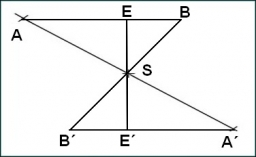Did you find an error or inaccuracy? Feel free to write us. Thank you!Tips to related online calculators
Check out our ratio calculator.
Do you want to convert length units?

#### You need to know the following knowledge to solve this word math problem:

We encourage you to watch this tutorial video on this math problem:

## Related math problems and questions:

• Similarity of two trianglesThe KLM triangle has a side length of k = 6.3cm, l = 8.1cm, m = 11.1cm. The triangle XYZ has a side length of x = 8.4cm, y = 10.8cm, z = 14.8cm. Are triangle KLM and XYZ similar? (write 0 if not, if yes, find and write the coefficient of a similarity)
• Rectangular trianglesThe lengths of corresponding sides of two rectangular triangles are in the ratio 2:5. At what ratio are medians relevant to hypotenuse these right triangles? At what ratio are the contents of these triangles? Smaller rectangular triangle has legs 6 and 8
• Similar trianglesThe triangles ABC and XYZ are similar. Find the missing lengths of the sides of the triangles. a) a = 5 cm b = 8 cm x = 7.5 cm z = 9 cm b) a = 9 cm c = 12 cm y = 10 cm z = 8 cm c) b = 4 cm c = 8 cm x = 4.5 cm z = 6 cm
• Sides od triangleSides of the triangle ABC has length 4 cm, 5 cm and 7 cm. Construct triangle A'B'C' that are similar to triangle ABC which has a circumference of 12 cm.
• Similarity coefficientThe ratio of similarity of two equilateral triangles is 3.5 (ie 7:2). The length of the side of smaller triangle is 2.4 cm. Calculate the perimeter and area of ​​the larger triangle.
• The triangles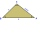The triangles KLM and ABC are given, which are similar to each other. Calculate the lengths of the remaining sides of the triangle KLM, if the lengths of the sides are a = 7 b = 5.6 c = 4.9 k = 5
• SimilarityABC is a triangle wherein a = 4 cm, b = 6 cm, c = 8 cm. Is it similar to the triangle DEF: d = 3 cm, e = 4.5 cm, f = 6 cm? If so, determine the ratio of similarity.
• SimilarityRectangle ABCD has dimensions of 7 cm and 6 cm. Rectangle PQRS has dimensions 14 cm and 12 cm. Determine coefficient of the similarity k of the rectangles, if they aren't similar enter zero as the coefficient of similarity.
• Two similar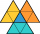Two similar triangles, one has a circumference of 100 cm, the second has sides successively 8 cm, 14 cm, 18 cm longer than the first. Find the lengths of its sides.
• Triangles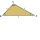Five sticks with a length of 2,3,4,5,6 cm. How many ways can you choose three sticks to form three sides of a triangle?
• Similar trianglesIn the triangle DEF is DE = 21cm, EF = 14.7cm, DF = 28cm. The triangle D´E´F´ is similar to the triangle DEF. Calculate the lengths of the sides of the triangle D´E´F´ if the similarity coefficient is one-seventh.
• 2d shapeCalculate the content of a shape in which an arbitrary point is not more than 3 cm from the segment AB. The length of the segment AB is 5 cm.
• Similarity coefficient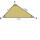The triangles ABC and A "B" C "are similar to the similarity coefficient 2. The sizes of the angles of the triangle ABC are α = 35° and β = 48°. Find the magnitudes of all angles of triangle A "B" C ".
• In aIn a triangle, the aspect ratio a: c is 3: 2, and a: b is 5: 4. The perimeter of the triangle is 74cm. Calculate the lengths of the individual sides.
• Similar trianglesTriangle A'B'C 'is similar to triangle ABC, whose sides are 5 cm, 8 cm, and 7 cm long. What is the length of the sides of the triangle A'B'C' if its circumference is 80 cm?
• Rhombus constructionConstruct ABCD rhombus if its diagonal AC=9 cm and side AB = 6 cm. Inscribe a circle in it touching all sides...
• Ratio of sidesThe triangle has a circumference of 21 cm, and the length of its sides is in a ratio of 6: 5: 3. Find the length of the longest side of the triangle in cm.